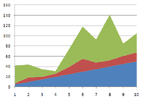###Teacher Guide to Graphing Resources

#### What are the different types of graphs commonly used? Why do we use them?

 Graphs, why do we use them? Graphs are commonly referred to as charts and are usually data which can be represented by symbols. A chart can easily represent numeric data and functions in order to give a person an idea of the current or even a forecast situation in a certain business or a schedule. Often in class we use graphing worksheets to help students understand the details of graphs. We hope to help you write fantastic graphing lesson plans with just a little extra knowledge. The commonly used graphs for data presentation are: Pictographs - This is the most basic form of graphing. This is usually where we start all students. As you can see below, a pictograph just display numerical information or data using pictures or icons as a reference.Reading and Creating Pictographs Worksheet Series This series helps students in identifying a pictograph legend and key, plotting pictographs, and understanding the reason for graphing this data. This series works students through a process to transition to bar graphs very quickly. Includes estimating with pictographs and predicting outcomes based on past data. View Lesson SetHistograms - This is a graph display of frequencies that appear as rectangles which show intervals where progression is measured over time. Histograms are used to present continuous data. For example, numbers of people who go to the shopping mall over the span of how many months, and so on.

Bar graphs - This is a chart that uses rectangular bars and the lengths along the y-axis are proportional to the quantity of whatever value it is they represent. The x-axis represents the period within which this value was measured. Even though bar graphs look similar to histograms, they are used to display information that is category centered or focused on different subjects. For example, if you were to take a class survey to find out students favorite subject area. The data might have five to ten different categories (Math, Science, Social Studies, English, Health, Physical Education, Economics, etc.)Reading and Creating Bar Graphs A great self guided series of lessons for students on bar graphs. It walks students through the process of identifying the axis, plotting points, connecting points, and making sense of the outcome of data that is graphed. This series works students through a process to transition to line graphs very quickly. Includes vertical and horizontal bar graphs. Provides plenty of guided and independent practice for creating and reading bar graphs. View Lesson Set

Pie charts - This is where the chart or graph is depicted as a round disc or pie that is divided into portions according to the percentages of values being represented. This concept is widely used by businesses when they make their budget studies and they wish to see the whole in proportion to their "slice" of the pie.Reading and Creating Pie Charts and Graphs This series walks students through the process of identifying a pie graph legend and key, creating pie graphs, and understanding the reason for graphing this data. Includes estimating with pie charts and predicting outcomes based on past data. View Lesson Set

Line chart or linear graph - This is a graph that measures a series of data points on y-axis over an x-axis of time. This is a point-point type of system where a period of time determines at which point the line or trend must end, and a new one begins.Reading and Creating Line Graphs Lesson Series Looks at the process of identifying the axis, plotting points, connecting points, and making sense of the outcome of data that is graphed. Includes estimating with line graphs and predicting outcomes based on past data. Provides plenty of guided and independent practice for creating and reading line graphs. View Lesson SetArea graphs - This is a graph that represents quantitative data and derives its basis from the line graph. The area between x-axis and the line are usually filled in so as to give an idea of cumulated totals or "stacks." This gives you a quick reference as to the relative characteristics of the data.

Coordinate Graphs - Simply put a coordinate graph demonstrates a relationship between numbers. A coordinate grid is composed of axes. The left to right (horizontal) axis is called the x-axis. The x-axis increases in value as you move to the right and decreases in value as you move to left. The up to down (vertical) axis is called the y-axis. The y-axis increases in value as you move up and decreases in value as you move down.Reading & Creating Coordinate Graphs This complete series will take students from beginner skills such as identifying the axis, plotting points, connecting points, and advance them through to making sense of the outcome of data that is graphed. View Lesson Set

Most graphs are used for presentation especially for businesses worldwide. This is used for their forecasting of sales, products, and budget over time. It really depends on what a presentation needs to be in order to determine what are the different types of graphs commonly used for certain studies and/or businesses.

There are so many other common and uncommon ways of graph presentations that can be used for giving an audience an idea of the current and possibly future goals a company may intend to achieve. This hopefully addresses the question of graphs and the question of why do we use them.